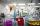# Square and rectangle

Calculate the side of a square which content area equals area of the rectangle having a length of 3 cm greater and by 2 cm smaller than the side of the square.

Result

a =  6 cm

#### Solution:

a^2 = (a+3)*(a-2)

a = 6

a = 6

Calculated by our simple equation calculator.

Leave us a comment of example and its solution (i.e. if it is still somewhat unclear...):

Showing 0 comments:Be the first to comment!#### To solve this example are needed these knowledge from mathematics:

Looking for help with calculating roots of a quadratic equation? Do you have a linear equation or system of equations and looking for its solution? Or do you have quadratic equation?

## Next similar examples:

1. Rectangle vs squareOne side of the rectangle is 1 cm shorter than the side of the square, the second side is 3 cm longer than the side of the square. Square and rectangle have the same content. Calculate the length of the sides of a square and a rectangle.
2. RectangleCalculate area of the rectangle if its length is 12 cm longer than its width and length is equal to the square of its width.
3. Rectangle - area, perimeterThe area of a rectangular field is equal to 300 square meters. Its perimeter is equal to 70 meters. Find the length and width of this rectangle.
4. Quadratic equationQuadratic equation ? has roots x1 = 80 and x2 = 78. Calculate the coefficients b and c.
5. Square root 2If the square root of 3m2 +22 and -x = 0, and x=7, what is m?
6. Solve equationsolve equation: ?
7. RootsDetermine the quadratic equation absolute coefficient q, that the equation has a real double root and the root x calculate: ?
8. Crystal waterThe chemist wanted to check the content of water of crystallization of chromic potassium alum K2SO4 * Cr2 (SO4) 3 * 24 H2O, which was a long time in the laboratory. From 96.8 g of K2SO4 * Cr2 (SO4) 3 * 24 H2O prepared 979 cm3 solution of base. S
9. EquationEquation ? has one root x1 = 8. Determine the coefficient b and the second root x2.
10. Evaluation of expressionsIf a2-3a+1=0, find (i)a2+1/a2 (ii) a3+1/a3
11. Solve 3Solve quadratic equation: (6n+1) (4n-1) = 3n2
12. DiscriminantDetermine the discriminant of the equation: ?
13. Quadratic equationFind the roots of the quadratic equation: 3x2-4x + (-4) = 0.
14. VariableFind variable P: PP plus P x P plus P = 160
15. Cinema 4In cinema are 1656 seats and in the last row are 105 seats , in each next row 3 seats less. How many are the total rows in cinema?
16. Expressions 3If k(x+6)= 4x2 + 20, what is k(10)=?
17. Theorem proveWe want to prove the sentense: If the natural number n is divisible by six, then n is divisible by three. From what assumption we started?